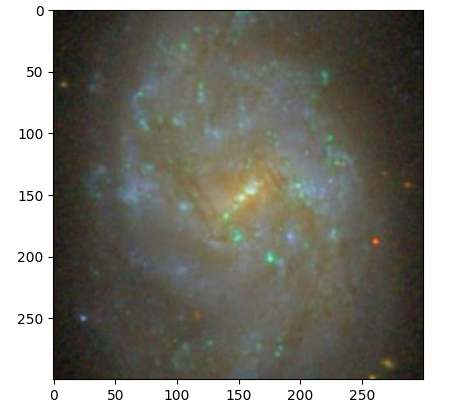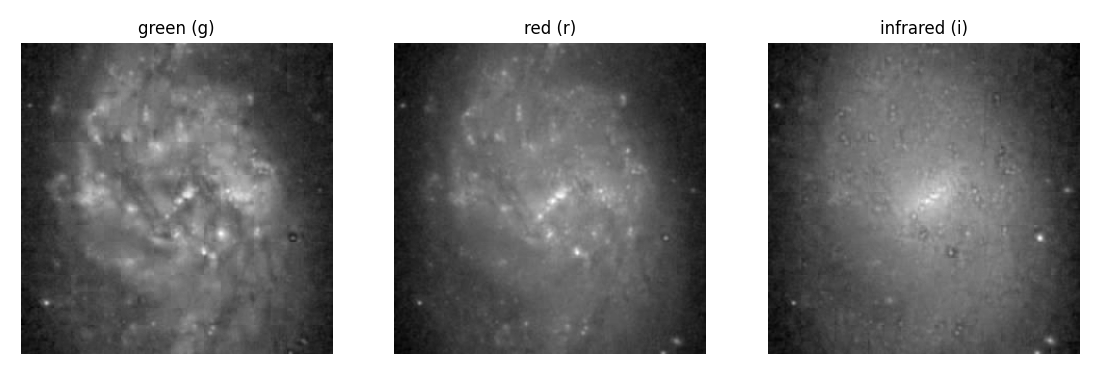# sdss

A python package for retrieving and analysing data from SDSS (Sloan Digital Sky Survey)

## Installation

``````pip install sdss
``````

Requirements are numpy, Pillow, matplotlib and pandas.

## Quick start

Let's get the positions of the sun between two times:

``````from sdss import Region

ra = 179.689293428354
dec = -0.454379056007667

img = Region(ra, dec)
``````

To see the image:

``````img.show()
``````To see the image in three gri filter bands (green, red, infrared) separately:

``````img.show3b()
``````To find 10 nearest objects in a radius of 5 arc degrees:

``````df_obj = img.nearest_objects(radius=5, n=10)
``````

To find 10 nearest objects with spectrum in a darius of 5 arc degrees:

``````df_sp = img.nearest_spects(radius=5, n=10)
``````

## GitHub

https://github.com/behrouzz/sdss